# 【Ray Tracing in One Weekend 超详解】 光线追踪1-9 景深

Chapter11：Defocus Blur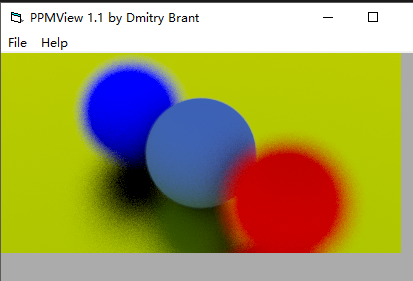Preface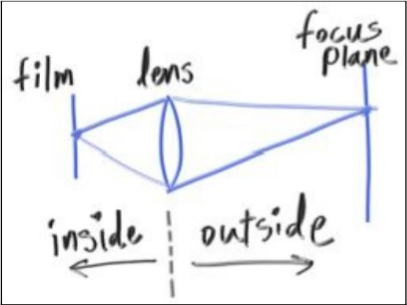正文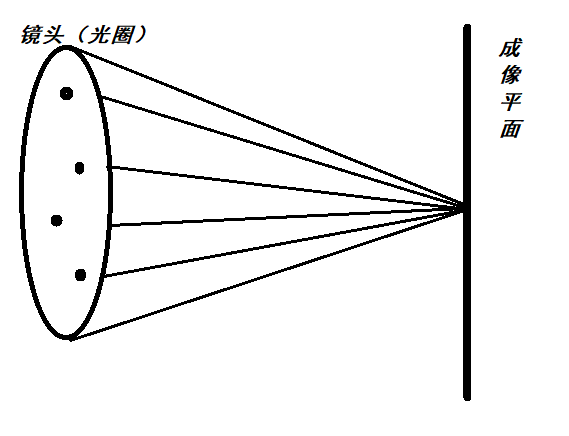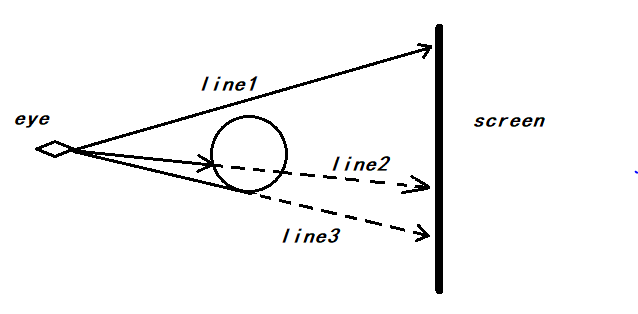(图片来自百度百科：)

随之，我们的成像平面也就到了z = -focus，或者是-focus * w平面(按w基向量算)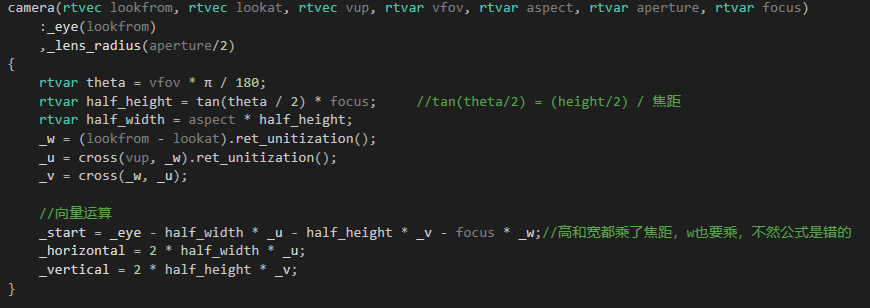const rtvec random_unit_disk()            //find a random point in unit_disk
{
rtvec p;
do
{
p = 2.0*rtvec(rtrand01(), rtrand01(), 0) - rtvec(1, 1, 0);
} while (dot(p, p) >= 1.0);
return p;
}/// camera.h

// -----------------------------------------------------
// [author]        lv
// [begin ]        2019.1
// [brief ]        the camera-class for the ray-tracing project
//                from the 《ray tracing in one week》
// -----------------------------------------------------

#ifndef CAMERA_H
#define CAMERA_H

#include "ray.h"

namespace rt
{

class camera
{
public:
camera(rtvec lookfrom, rtvec lookat, rtvec vup, rtvar vfov, rtvar aspect, rtvar aperture, rtvar focus)
:_eye(lookfrom)
{
rtvar theta = vfov * π / 180;
rtvar half_height = tan(theta / 2) * focus;        //tan(theta/2) = (height/2) / 焦距
rtvar half_width = aspect * half_height;
_w = (lookfrom - lookat).ret_unitization();
_u = cross(vup, _w).ret_unitization();
_v = cross(_w, _u);

//向量运算
_start = _eye - half_width * _u - half_height * _v - focus * _w;//高和宽都乘了焦距，w也要乘，不然公式是错的
_horizontal = 2 * half_width * _u;
_vertical = 2 * half_height * _v;
}

inline const ray get_ray(const rtvar u,const rtvar v)const
{
rtvec rd = _lens_radius * random_unit_disk();
rtvec offset = _u * rd.x() + _v * rd.y();
return ray{ _eye + offset, _start + u*_horizontal + v*_vertical - (_eye + offset) };
}

inline const ray get_ray(const lvgm::vec2<rtvar>& para)const
{    return get_ray(para.u(), para.v());    }

inline const rtvec& eye()const { return _eye; }

inline const rtvec& start()const { return _start; }

inline const rtvec& horizontal()const { return _horizontal; }

inline const rtvec& vertical()const { return _vertical; }

inline const rtvec& u()const { return _u; }

inline const rtvec& v()const { return _v; }

inline const rtvec& w()const { return _w; }

inline const rtvar lens_r()const { return _lens_radius; }

private:
rtvec _u;

rtvec _v;

rtvec _w;

rtvec _eye;

rtvec _start;        //left-bottom

rtvec _horizontal;

rtvec _vertical;

};

}

#endif
camera.h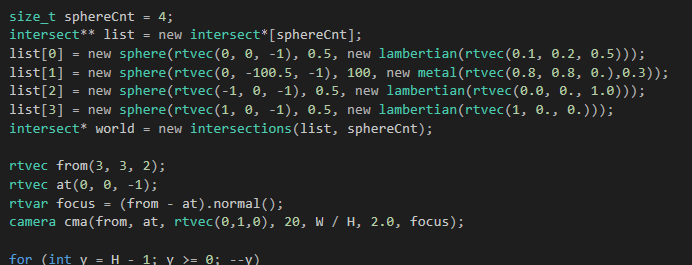晚安

posted @ 2019-01-05 01:49  林-兮  阅读(...)  评论(... 编辑 收藏